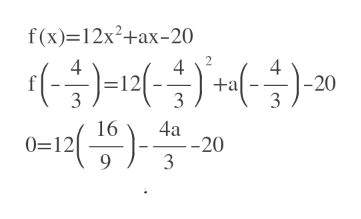# In the expression below, a is an integer. 12x2 + ax -20 If 3x +4 is a factor of the expression above what is the value of a?

Question

In the expression below, a is an integer.

12x2 + ax -20

If 3x +4 is a factor of the expression above what is the value of a?

check_circleExpert Solution
Step 1

3x+4 is a factor so by factor theorem ,

3x+4=0

x= -4/3 is a zero of the equation.

Step 2

f(-4/3) =0, because x=-4/3 is a zero of f(x).

Using this we ...help_outlineImage Transcriptionclosef(x) 12x2+ax-20 4 -20 3 - 12 | +a 3 3 16 0=12 9 4a -20 3 fullscreen

### Want to see the full answer?

See Solution

#### Want to see this answer and more?

Solutions are written by subject experts who are available 24/7. Questions are typically answered within 1 hour*

See Solution
*Response times may vary by subject and question
Tagged in

### Other